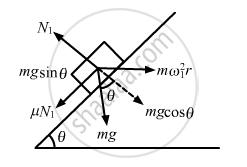Share

# A Hemispherical Bowl of Radius R is Rotated About Its Axis of Symmetry Which is Kept Vertical. a Small Block is Kept in the Bowl at a Position Where the Radius Makes an Angle θ with the Vertical. - Physics

ConceptCircular Motion

#### Question

A hemispherical bowl of radius R is rotated about its axis of symmetry which is kept vertical. A  small block is kept in the bowl at a position where the radius makes an angle θ with the vertical. The  block rotates with the bowl without any slipping. The friction coefficient between the block and the bowl surface is μ. Find the range of the angular speed for which the block will not slip.

#### Solution

When the bowl rotates at maximum angular speed, the block tends to slip upwards.
Also, the frictional force acts downward.
Here, we have:
Radius of the path that the block follow = r = Rsinθ
Let N1 be the normal reaction on the block and ω1 be the angular velocity after which the block will slip.
From the free body diagram-1, we get :

$N_1 - \text{mg}\cos\theta = {m\omega}_1^2 (R\sin\theta)\sin\theta . . .$(i)

$\mu N_1 + \text{mg}sin\theta = m \omega_1^2 (R\sin\theta)\cos\theta . . .$(ii)On solving the two equation, we get :

$\omega_1 = \left[ \frac{g(\text{sin}\theta + \mu\text{ cos}\theta)}{\text{R sin}\theta (\text{cos}\theta - \mu \text{ sin }\theta)} \right]^{1/2}$

Let us now find the minimum speed (ω2) on altering the direction of $\mu$ (as shown in figure):

$\omega_2 = \left[ \frac{g(\text{ sin }\theta - \mu \text{ cos }\theta)}{\text{R sin}\theta (\text{ cos }\theta + \mu \text{ sin }\theta)} \right]^{1/2}$Hence, the range of speed is between ω2 and ω1.

Is there an error in this question or solution?

#### APPEARS IN

Solution A Hemispherical Bowl of Radius R is Rotated About Its Axis of Symmetry Which is Kept Vertical. a Small Block is Kept in the Bowl at a Position Where the Radius Makes an Angle θ with the Vertical. Concept: Circular Motion.
S